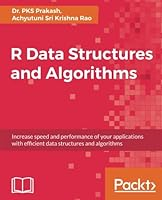# R Data Structures and Algorithms## Book Description

Increase speed and performance of your applications with efficient and algorithms About This Book See how to use such as arrays, stacks, trees, lists, and graphs through real-world examples Find out about important and advanced such as searching and sorting algorithms Understand important concepts such as big-o notation, programming, and functional data structured Who This Book Is For This book is for R developers who want to use data structures efficiently. Basic knowledge of R is expected. What You Will Learn Understand the rationality behind data structures and algorithms Understand computation evaluation of a program featuring asymptotic and empirical Get to know the of arrays and linked-based data structures Analyze types of sorting algorithms Search algorithms along with hashing Understand linear and tree-based indexing Be able to implement a including topological sort, shortest path problem, and Prim’s algorithm Understand dynamic programming (Knapsack) and randomized algorithms In Detail In this book, we cover not only classical data structures, but also functional data structures. We begin by answering the fundamental question: why data structures? We then move on to cover the relationship between data structures and algorithms, followed by an analysis and evaluation of algorithms. We introduce the fundamentals of data structures, such as lists, stacks, queues, and dictionaries, using real-world examples. We also cover topics such as indexing, sorting, and searching in depth. Later on, you will be exposed to advanced topics such as data structures, dynamic programming, and randomized algorithms. You will come to appreciate the intricacies of high performance and scalable programming using R. We also cover special R data structures such as vectors, data frames, and atomic vectors. With this easy-to-read book,

Chapter 1. Getting Started
Chapter 2. Algorithm Analysis
Chapter 4. Stacks and Queues
Chapter 5. Sorting Algorithms
Chapter 6. Exploring Search Options
Chapter 7. Indexing
Chapter 8. Graphs
Chapter 9. Programming and Randomized Algorithms
Chapter 10. Functional Data Structures